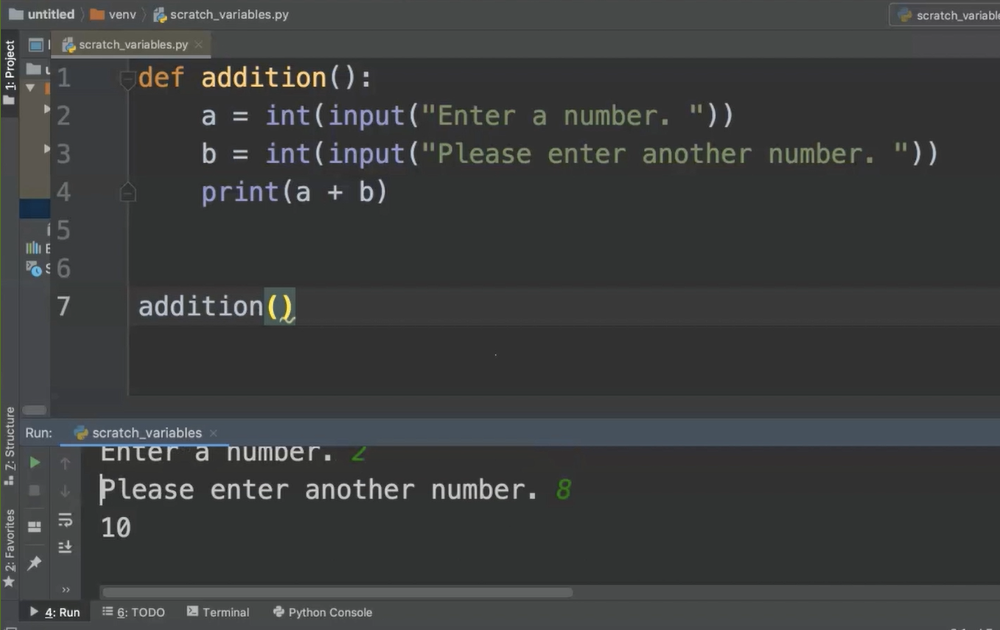Transcripted Summary

In this chapter we'll be learning about functions.

Before we make functions, we need to figure out what is a function.

### # What is a Function?

A function is a unit of code that can be reused throughout a program.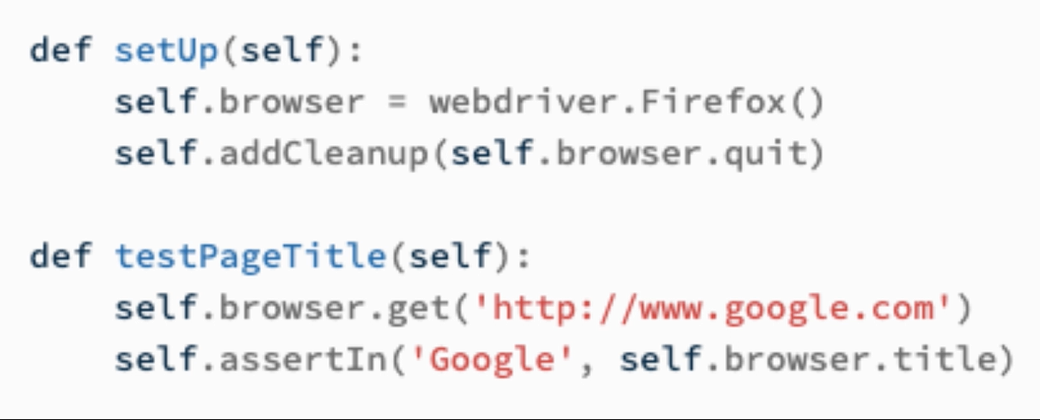You'll notice that the 2 functions in this example contain several lines of code.

Each function can be called and used by itself, and yet we get the benefit of every line of code that's inside of that function.

Functions provide us re-usability and modularity throughout our code.

Now, we'll learn how functions are built.

### # Parts of a Function

On every function, we have the letters `def`, a unique function name, the parenthesis and the colon.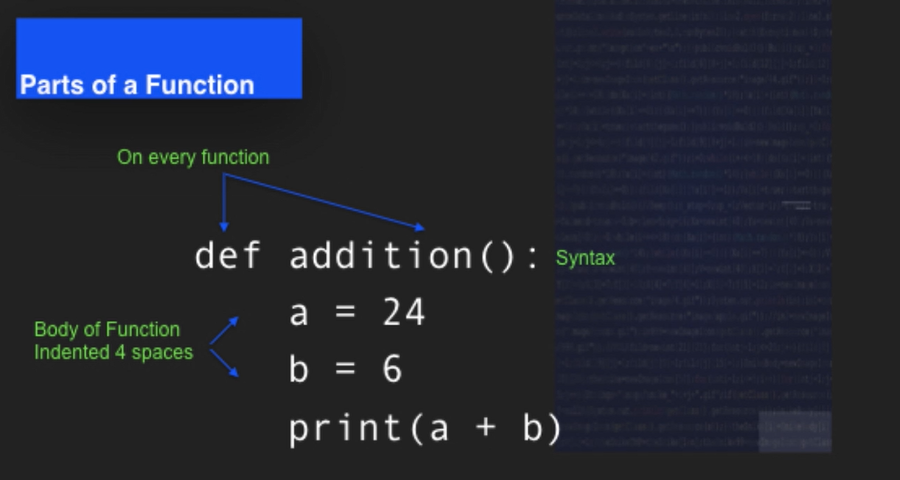The body of our function is always indented 4 spaces and we remove the spaces when we want to call a function.

We'll use the unique name and the parenthesis to call the function.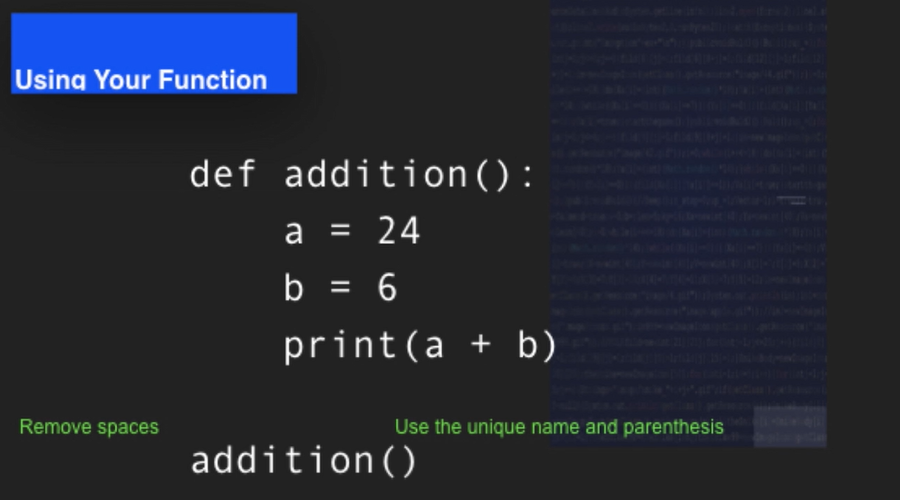Let's go ahead and try this out in the IDE.

We'll go ahead and get started by creating a basic Python function.

Every Python function begins with `def`.

For this video, we'll work with a basic addition function and “addition” is our unique name for the function in our program.

Any unique function name needs to be followed up by parenthesis.

In Python, those parentheses can accept arguments and we'll learn more about that a little bit later on. But right now, we'll just keep those parentheses empty and they always need to be there.

We'll end the first line of our function with a colon.

When I hit return, you'll notice that the IDE automatically indents, and that indent is 4 spaces.

REMINDER

If you are not working in PyCharm, you will want to make sure that every time you indent, the indent is equivalent to 4 spaces. White space is part of how Python helps to organize the code.

I will set variable “a” equal to 10.

I'll set a second variable “b” equal to 30.

Since this is a lot like a math function, I'll go ahead and use these variables just like math class and have my output as print a plus b.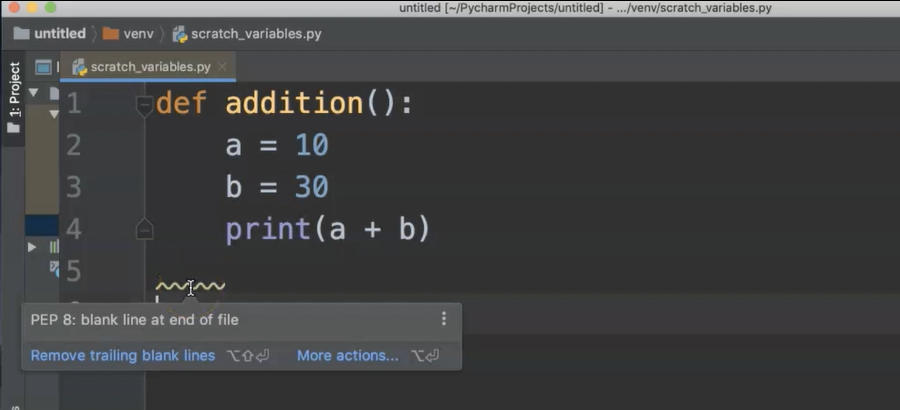This seems simple.

You'll notice that lines come up when there are things that the IDE considers an error. Some of these are actual problems.

Others are PEP 8 issues which are formatting issues. As we are moving through and programming in a more sketch-like fashion where we're just trying things out, it's okay to let some of the PEP 8 errors go for now.

When you are writing programs in a more official capacity for work or to do automation, then you want to make sure that your code is conforming to PEP 8 and utilize the PEP 8 standards more often.

But for our purposes, it's okay if these things show up.

What I do want to do is go ahead and go to the Run tab.

Run and then select my `scratch_variables.py` file.

It ran so fast but there is no output and we have a print statement.

What happened?

Well, when we have a function in Python, we also have to call that function.

In order to do that, we need to do 2 things.

1 - We need to write the unique name of the function.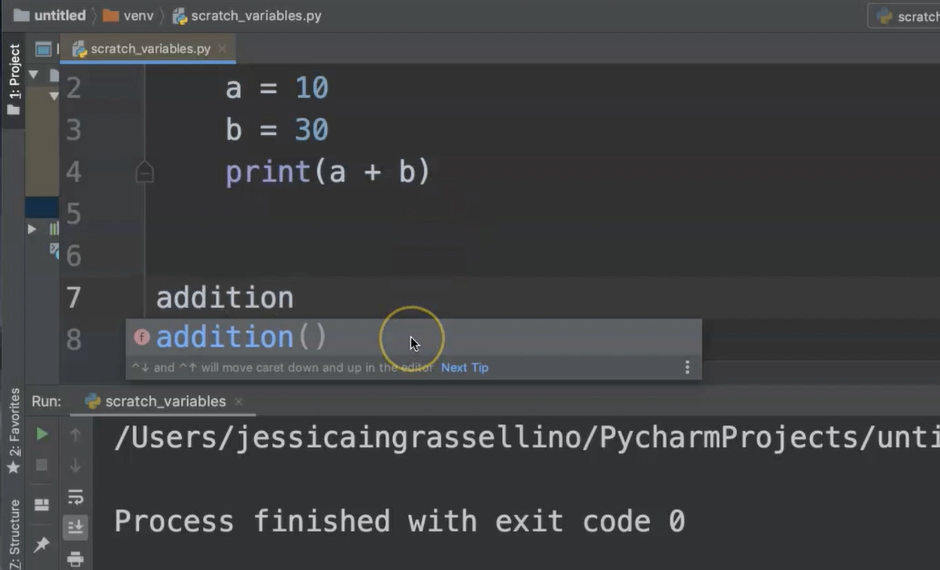You'll notice our custom functions come up and we can either type it out completely or we can click, hit enter and the function will be completed.

2 - You have the unique name followed by the parenthesis.

``````def addition():
a = 10
b = 30
print(a + b)

``````

When you rerun the code, now you have the function output of 40.

This is how a basic function works.

Sometimes, even when I'm writing simple code, I like to double check that the code is working. I might put something else in just to make sure that I am getting similar results that I expect.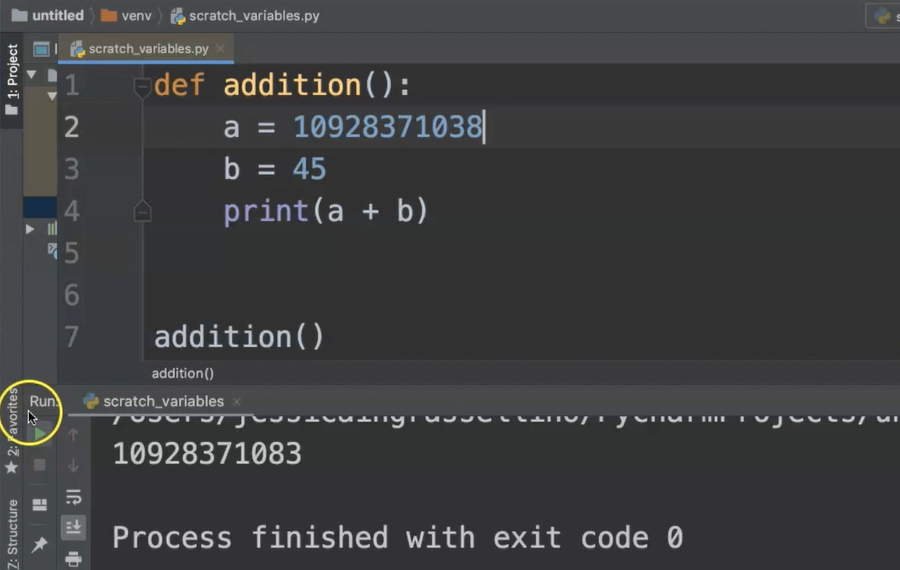Sometimes, I'll try something ridiculous, a little bigger and then I might try different kinds of data.

These are all just happy path tests to make sure that my code is doing the thing I expect it to do.

Right now, it is.

So, this is your overview of a basic function and how it works in Python.

### # Getting User Input

We have our addition function, but what we don't have is a way to actually flexibly get user input. It's not like a real calculator.

This is where our Python user `input` function can come in.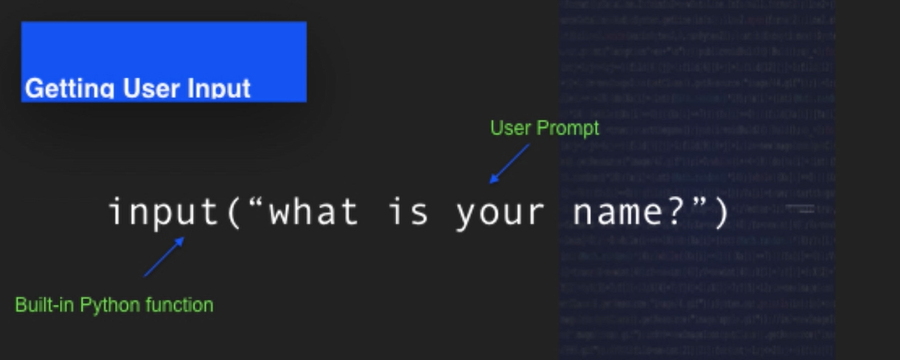We have the `input` function and in the parenthesis, we have the user prompt.

The user prompt is a question or a direction that we would like to give the user so that way, they give us the information that we need to run the program.

This prompt is always a String; and the input that goes into this prompt is also a String, so we'll need to do a little bit of magic with it to make it work in our calculator.

You'll notice that I've actually converted the String data into an integer by using `int` and wrapping that `input` function into an integer function.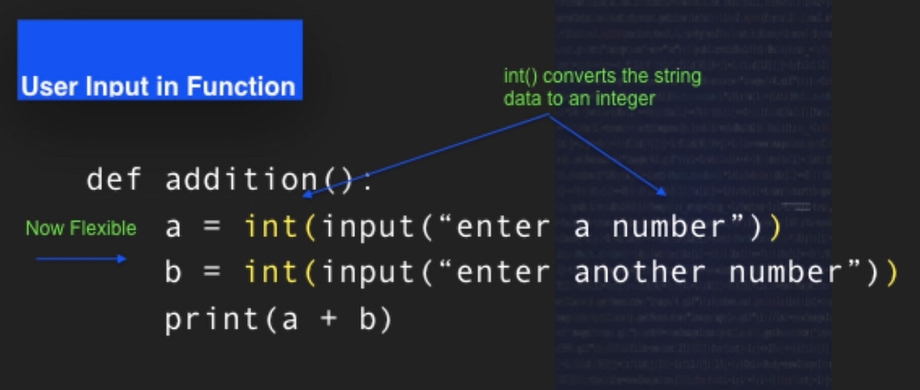That converts the String data that's taken from the user into an integer that we can use in a mathematical formula.

Now, we have a flexible way to get information from our users and use it in a program.

### # Apply `input()`

We'll go back to our `addition` function and we'll replace our variables where we've added numbers with our input function.

First, we're going to use our `int()` function, which is going to convert the data that the user enters into an integer.

Then, we're going to use our `input` function and you're going to use our second set of parentheses and we're going to give our user a prompt, “Enter a number”.

We'll go ahead and do the same thing with “b” changing our hard-coded variable into integer.

Convert `input`, “Please enter another number”.

I'm going to go ahead and make sure that it looks good and I'll add a space here so that way that when it prints on the screen, there'll be a space between the end of the sentence and where the user actually enters their input.

And then, I'll go ahead and skip two lines.

That's the Python standard is to put 2 lines between the end of your function and the beginning of your next one.

I will call the function by writing the unique name of the function, parenthesis.

``````def addition():
a = int(input("Enter a number. "))
b = int(input("Please enter another number. "))
print(a + b)

``````

I'll go up to Run.

I will select Run and now I'm running a file called `scratch_variables.py`.

As soon as I run that file, I have my space, which is great.

• “Enter a number. ” — and I'll enter 2.

• “Please enter another number. “ — go ahead and enter 8.

And you'll notice my outcome is 10, exit code zero.